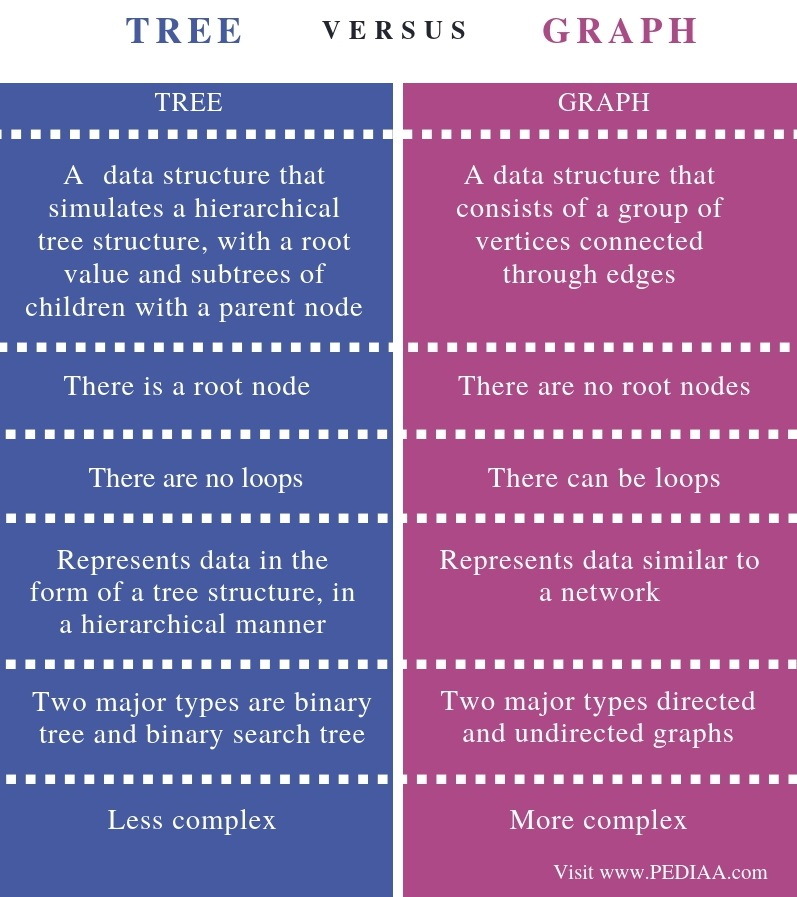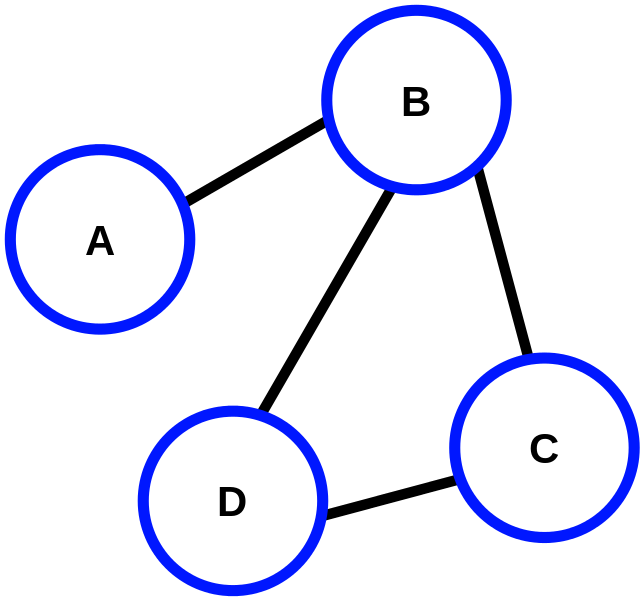What is the Difference Between Tree and Graph

The main difference between tree and graph is that a tree organizes data in the form of a tree structure in a hierarchy while a graph organizes data as a network.

A data structure is a way of organizing data in a systematic way. There are mainly two types of data structures as linear data structures and nonlinear data structures. And, the two common nonlinear data structures are tree and graph.

Key Areas Covered

1. What is a Tree
– Definition, Functionality
2. What is a Graph
– Definition, Functionality
3. What is the Difference Between Tree and Graph
– Comparison of Key Differences

Key Terms

Binary Search, Graph, Linear Data Structures, Nonlinear Data Structures, TreeWhat is a Tree

A tree is a data structure that arranges the data similar to a tree. A node is a data item in the tree. The major node is the root, and the other nodes are its children nodes. All these other nodes are arranged into the non-empty sets where each one of them is a subtree. Moreover, there is a parent-child relationship between the nodes. One parent node can have multiple child nodes, and there can only be one parent node for each child node.Some important terms related to a tree are as follows. You can see these features and examples in the above tree.

Root node is the topmost data item in the tree. Element 8 is the root node in the above image.

Edge helps to connect nodes. For example, in the above tree, edges connect 8 and 3, 8 and 10.

Parent node is a node other than the root node that connects upwards by an edge. For instance, 3 is the parent node of 1 and 6. Similarly, 6 is the parent node of 4 and 7.

Child node is a node that connects downwards by an edge. For example, 4 and 7 are child nodes of 6.

Leaf node is a node that does not have any child nodes. 1, 4,7,13 are leaf nodes in the above tree.

Subtree is a descendant of a node. For example, the section to the left of the root node (8) that begins from 3 is a subtree. Similarly, the section to the right of the root node that begins from 10 is a subtree.

Level represents the generation of nodes. As an example, the root node belongs to level 0. 3 and 10 belongs to level 1 and so on.

Furthermore, there are two major tree types as binary tree and binary search tree. In a binary tree, each node can have a maximum of 2 child nodes. A binary search tree is an ordered binary tree.

What is a Graph

A graph is a data structure that represents a pictorial structure of a set of objects that connects some pairs of objects by links. Usually, graphs help to represent networks.Some important terms related to the graph are as follows.

Vertices are objects or the data items. The circles represent them. In the above graph, A, B, C, and D are vertices. We can also write vertices as V = {A,B,C,D}.

Edges are the links that connect the vertices. For example, edges above connect A and B vertices, B and D vertices, etc. We can also write edges as E = {AB, BC, BD, DC}

Path represents the sequence of nodes to follow in order to reach the destination node. For example, ABD represents the path from vertex A to D.

When two nodes connect to each other through an edge, they are adjacent nodes. For instance, A and B are adjacent nodes. Similarly, B and D are adjacent nodes.

The major operations we can perform on graphs are adding vertexes, adding edges and displaying vertexes.

Mainly, there are two types of graphs as directed and undirected graphs. When a graph contains an ordered pair of vertices, it is a directed graph, and when a graph contains an unordered pair of vertices, it is an undirected graph.

Difference Between Tree and Graph

Definition

A tree is a data structure that simulates a hierarchical tree structure, with a root value and subtrees of children with a parent node whereas a graph is a data structure that consists of a group of vertices connected through edges. Thus, this is the fundamental difference between tree and graph.

Types

Also, the two major types of trees are binary tree and binary search tree. Whereas, the two major types of graphs are directed and undirected graphs.

Data representation

A tree represents data in the form of a tree structure, in a hierarchical manner, while a graph represents data similar to a network. Hence, this is the main difference between tree and graph.

Root node

Furthermore,  one other major difference between tree and graph is that there is a root node in the tree while there are no root nodes in a graph.

Loops

Moreover, the presence of loops is another difference between tree and graph. There are no loops in a tree while there can be loops in a graph.

Complexity

Besides, a graph is more complex than a tree.

Conclusion

Tree and graph are two nonlinear data structures. The main difference between tree and graph is that a tree organizes data in the form of a tree structure in a hierarchy while a graph organizes data as a network.

Reference:

1. “Tree – Javatpoint.” Www.javatpoint.com, Available here.
2. “DS Graph – Javatpoint.” Www.javatpoint.com, Available here.
3. “Graphs in Data Structure”, Data Flow Architecture, Available here.
4. “Tree (Data Structure).” Wikipedia, Wikimedia Foundation, 15 Jan. 2019, Available here.

Image Courtesy:

1. “Binary search tree” By Dcoetzee assumed – Own work assumed (based on copyright claims), (Public Domain) via Commons Wikimedia
2. “CPT-Graphs-undirected-unweighted-ex1” By Pluke – Own work (CC0) via Commons Wikimedia